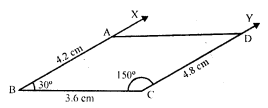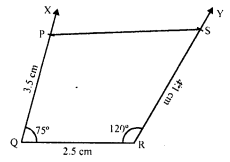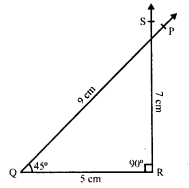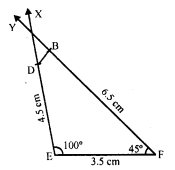## RD Sharma Class 8 Solutions Chapter 18 Practical Geometry Ex 18.4

These Solutions are part of RD Sharma Class 8 Solutions. Here we have given RD Sharma Class 8 Solutions Chapter 18 Practical Geometry Ex 18.4

Other Exercises

Question 1.
Construct a quadrilateral ABCD, in which AB = 6 cm, BC = 4 cm, CD = 4 cm, ZB = 95° and ∠C = 90°.
Solution:
Steps of construction :
(i) Draw a line segment BC = 4 cm.(ii) At B, draw a ray BX making an angle of 95° and cut off BA = 6 cm.
(iii) At C, draw a ray CY making an angle of 90° and cut off CD = 4 cm.
Then ABCD is the required quadrilateral.

Question 2.
Construct a quadrilateral ABCD, where AB = 4.2 cm, BC = 3.6 cm, CD = 4.8 cm, ∠B = 30° and ∠C = 150°.
Solution:
Steps of construction :
(i) Draw a line segment BC = 3.6 cm.
(ii) At B, draw a ray BX making an angle of 30° and cut of BA = 4.2 cm.
(iii) At C, draw another ray CY making an angle of 150° and cut off CD = 4.8 cm.Then ABCD is the required quadrilateral.

Question 3.
Construct a quadrilateral PQRS, in which PQ = 3.5 cm, QR = 2.5 cm, RS = 4.1 cm, ∠Q = 75° and ∠R = 120°.
Solution:
Steps of construction :
(i) Draw a line segment QR = 2.5 cm.(ii) At Q, draw a ray QX making an angle of 75° and cut off QP = 3.5 cm.
(iii) At R, draw another ray RY making an angle of 120° and cut off RS = 4.1 cm.
(iv) Join PS.
Then PQRS is the required quadrilateral.

Question 4.
Construct a quadrilateral ABCD given BC = 6.6 cm, CD = 4.4 cm, AD = 5.6 cm and ∠D = 100° and ∠C = 95°.
Solution:
Steps of construction :
(i) Draw a line segment CD = 4.4 cm.(ii) At C, draw a ray CX making an angle of 95° and cut off CB = 6.6 cm
(iii) At D, draw another ray DY making an angle of 100° and cut off DA = 5.6 cm.
(iv) Join AB.
Then ABCD is the required quadrilateral.

Question 5.
Construct a quadrilateral ABCD in which AD = 3.5 cm, AB = 4.4 cm, BC = 4.7 cm, ∠A = 125° and ∠B = 120°.
Solution:
Steps of construction :
(i) Draw a line segment AB 4.4 cm.(ii) At A, draw a ray AX making an angle of 125° and cut off AD = 3.5 cm.
(iii) At B, draw another ray BY making an angle of 120° and cut off BC = 4.7 cm.
(iv) Join CD.
Then ABCD is the required quadrilateral.

Question 6.
Construct a quadrilateral PQRS in which ∠Q = 45°, ∠R = 90°, QR = 5 cm, PQ = 9 cm and RS = 7 cm.
Solution:
Steps of construction :
This quadrilateral is not possible to construct as shown in the figure.Question 7.
Construct a quadrilateral ABCD in which AB = BC = 3 cm, AD = 5 cm, ∠A = 90° and ∠B = 105°.
Solution:
Steps of construction :
(i) Draw a line segment AB = 3 cm.(ii) At A, draw a ray AX making an angle of 90° and cut off AD = 5 cm.
(iii) At B, draw another ray BY making an angle of 105° and cut off BC = 3 cm.
(iv) Join CD.
Then ABCD is the required quadrilateral.

Question 8.
Construct a quadrilateral BDEF where DE = 4.5 cm, EF = 3.5 cm, FB = 6.5 cm and ∠F = 50° and ∠E = 100°
Solution:
Steps of construction :
(i) Draw a line segment EF = 3.5 cm.
(ii) At E, draw a ray EX making an angle of 100° and cut off ED = 4.5 cm.
(iii) At F, draw another ray FY making an angle of 45° and cut off FB = 6.5 cm.
(iv) Join DB.Then BDEF is the required quadrilateral.

Hope given RD Sharma Class 8 Solutions Chapter 18 Practical Geometry Ex 18.4 are helpful to complete your math homework.

If you have any doubts, please comment below. Learn Insta try to provide online math tutoring for you.